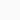# Angular Acceleration: Formula Concept UnitBy: Stupid
Updated:
Physics

Angular acceleration: The time rate of change of angular velocity. The common unit is angle per second squared.

## Angular Acceleration: Theory

Definition of Angular Acceleration: The rate of change of angular velocity with respect to time is called angular acceleration. Alternatively, the change of angular velocity per unit of time is also called angular acceleration.

Let us consider, that the initial angular velocity of a particle is ω1. After time t its final angular velocity is ω2. Therefore the change in angular velocity is Δω = ω2 − ω1. So, by definition –

$$Angular\:acceleration (\alpha ) = \frac{Change\:in\:angular\:velocity }{time(t)}$$
$$\overrightarrow{\alpha } = \frac{\Delta \overrightarrow{\omega }}{t} = \frac{\overrightarrow{\omega _{2}}- \overrightarrow{\omega _{1}}}{t}$$

Instantaneous Angular Acceleration: It is the ratio of instantaneous change of angular velocity (Δω) to the infinitesimal change of time (Δt).Mathematically,

$$\mathrm{\alpha = \displaystyle \lim_{\Delta t \to 0} \frac{\Delta \omega }{\Delta t} = \frac{d\omega }{dt}}$$

Now,

$$\mathrm{\mathrm{\alpha = \frac{d\omega }{dt}} = \frac{\mathrm{d} }{\mathrm{d} t}(\frac{d\theta }{dt}) = \frac{\mathrm{d^{2}\theta } }{\mathrm{d} t^{2}}}$$

Where θ = angular displacement.

If the angular velocity of an object continues to increase, then the motion of the object consists of ‘angular acceleration’. When an electric fan is switched on, the speed of the fan consists of angular acceleration until it reaches maximum speed. Again, if the angular velocity of an object is constantly decreasing, its motion has ‘angular deacceleration’. In the case of angular deceleration, the value of the final angular velocity ω2 is less than the initial angular velocity ω1. As a result, ω2 – ω1 = α = a negative quantity. That is, angular deceleration is only negative angular acceleration.

### What is the direction of angular acceleration?

Angular acceleration is an axial vector. The direction of angular velocity and angular acceleration may be different. The direction of positive angular acceleration is the direction of angular velocity. But if the angular acceleration is negative, that is, when the angular deceleration occurs, the direction of angular velocity and angular acceleration is opposite to each other.

Angular acceleration is a vector quantity. Its direction is along the direction of angular velocity. Here in this article, one can find the direction of angular velocity.

Alert!! In two-dimensional space, angular acceleration is a pseudoscalar. In three-dimensional space, angular acceleration is a pseudovector.

## Unit and Dimension of Angular Acceleration:

The unit of angular acceleration is radian/second2.

Dimension of angular acceleration =

$$\frac{dimension\: of\:angular\:velocity}{Dimension\:of\:time}$$
$$= \frac{[T^{-1}]}{[T]}= [T^{-2}]$$

## Relation between Linear acceleration and angular acceleration:

Let us consider a particle rotating along a circular path of radius r. Also, its initial linear velocity is v1 and angular velocity ω1. After time t its linear velocity and angular velocity become v2 and ω2 respectively.

So, v1 = ω1r and v2 = ω2r (Since linear velocity = angular velocity × radus).

If f represents the magnitude of linear acceleration then –

$$f=\frac{v_{2} – v_{1}}{t}$$
$$or,\: f= \frac{\omega _{2}r – \omega _{1}r}{t}$$
$$or,\: f= r\times \frac{\omega _{2} – \omega _{1}}{t}$$

Again, we know that the angular acceleration α

$$\alpha= \frac{\omega _{2}- \omega _{1}}{t}$$
$$\therefore\: f = r\times \alpha$$

Linear Acceleration = Angular Acceleration × Radius of circle

The Vector relation of linear and angular acceleration is given below.

$$\therefore\: \overrightarrow{f} = \overrightarrow{r}\times \overrightarrow{\alpha}$$

## Angular Acceleration: Example Practice:

Exercise-1: An electric fan is rotating at 210 rpm. By using a regulator, its motion is increased to 630 rpm in 11 seconds. What is the angular acceleration of the electric fan?

The initial velocity ω1 = 210 × (2π/60) = 7π rad/sec.
The final velocity ω2 = 630 × (2π/60) = 21π rad/sec.

Now the angular acceleration α = (ω2−ω1)/t
or, α = (21π − 7π)/11 = 14π/11
or, α = (14/11) × ((22/7) = 4 rad/sec2.

Exercise-2: The angular displacement of a particle is θ = 2πt3 + πt2 + 4t − 5. Calculate its angular acceleration at time t = 10 seconds.

We have angular displacement θ = 2πt3 + πt2 + 4t − 5.

So the angular acceleration is given by the following equation.

$$\alpha = \frac{d^{2}\theta }{dt^{2}}$$
$$or,\: \alpha = \frac{\mathrm{d} }{\mathrm{d} t}(\frac{\mathrm{d}\theta }{\mathrm{d} t})$$
$$or,\: \alpha = \frac{\mathrm{d} }{\mathrm{d} t}(6\pi t^{2}+2\pi t+4)$$
$$or,\: \alpha = 12\pi t+2\pi$$

So, the angular acceleration at time t =10 seconds is –

$$or,\: \alpha|_{t=10} = 12\pi \times 10+2\pi$$
$$or,\: \alpha|_{t=10} = 122\pi\:rad/sec^{2}$$

Therefore, the angular acceleration of the particle at time t = 10 seconds is 122π rad/sec2.

Exercise-3: A cycle moves at a constant speed of 8 m/s in a circular path of radius of 2 m. What is the angular acceleration of the object?

We know that the relationship between angular and linear acceleration is f = αr. where f = linear acceleration and α = angular acceleration and r = radius.

Now the lnear acceleration = centripetal acceleration = f = v2/r = (8)2/2 = 32 m/s2 .

Therefore the angular acceleration α = f/r = 32/2= 16 rad/s2